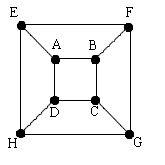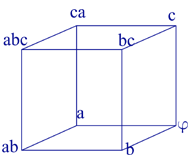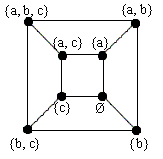#### You may also like### Instant Insanity

Given the nets of 4 cubes with the faces coloured in 4 colours, build a tower so that on each vertical wall no colour is repeated, that is all 4 colours appear.### Tree Graphs

A connected graph is a graph in which we can get from any vertex to any other by travelling along the edges. A tree is a connected graph with no closed circuits (or loops. Prove that every tree has exactly one more vertex than it has edges.### Magic Caterpillars

Label the joints and legs of these graph theory caterpillars so that the vertex sums are all equal.

# Cube Net

##### Age 16 to 18 Challenge Level:

Congratulations Andrei, School No. 205, Bucharest, Romania on another excellent solution.

1. I observed that a cube could be represented by the diagram below, that keeps all edges and vertices (the lengths are not important).From the beginning I observe that one could start from any vertex in a cube and there are $8$ vertices.

From any vertex there are three possible routes. I shall consider the routes starting from vertex $A$.

Below are written all the possible combinations I found starting with the edge $AD$:

 Path Circuit A-D-C-B-F-E-H-G No A-D-C-B-F-G-H-E-A Yes A-D-C-G-H-E-F-B-A Yes A-D-C-G-F - impossible A-D-H-E-F-B-C-G No A-D-H-E-F-G-C-B-A Yes A-D-H-G-C-B-F-E-A Yes A-D-H-G-F-E - impossible

I observe that there are $6$ possible paths, $4$ of which are Hamiltonian Circuits.

I have to multiply the number of solutions I obtained. So the total number of solutions is given by $8 \times3 \times6$ that is $144$, and $96$ are Hamiltonian Circuits.

Because all vertices of the cube are indistinguishable, there are $18$ solutions, and $12$ Hamiltonian Circuits.

2. Set $\{a, b, c\}$ has the following subsets: $\{a, b, c\}, \{a, b\}, \{a, c\}, \{b, c\}, \{a\}, \{b\}, \{c\}, \phi$. I observe that they could be arranged so that one subset is connected with $3$ other subsets that differ from the first by only one element, deleted or inserted. These subsets can be positioned on the vertices of a cube.I have verified that each subset is connected with $3$ other subsets, forming a diagram as found before, or, more intuitively, a cube. So, the problem is reduced to the first problem.

The number of sequences is $144$ sequences, or $18$ if the first element of the sequence does not matter.